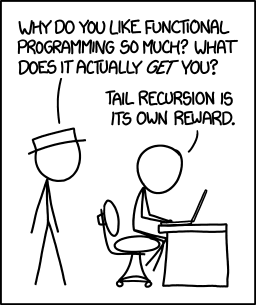Thanks again, Randall, for a stunningly amusing comic:### hover comment:

Functional programming combines the flexibility and power of abstract mathematics with the intuitive clarity of abstract mathematics.

Tail Recursion is a computer science term that refers to a particular form a recursion, where the recursive call is made at the end of the routine to itself. Functional programming languages such as Lisp and derivatives, and OO languages such as Ruby, use this to great effect.

The comment on the cartoon above, by Randall Monroe, provides the usual level of snark that we all must give ourselves for our vast pretentiousness. :)

The classic tail recursion example is calculating the factorial of a number, which is the formula:And below is how it is implemented in Common Lisp:

``````(defun factorial (n)
(if (= n 0)
1
(* n (factorial (- n 1)))
)
)
``````